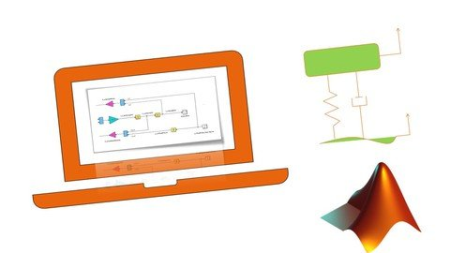# Hello Guest! Welcome to my website.

You can benefit from our privileges as a member of our site.

#### 0nelove

##### Active memberGenre: eLearning | MP4 | Video: h264, 1280x720 | Audio: AAC, 48.0 KHz
Language: English | Size: 3.01 GB | Duration: 7h 38mGet familiar with Simulink - MATLAB/SIMULINK, PID, Fuzzy Logic Control, 1 and 2 Degree of Freedom Vibration/Suspension

What you'll learn
Familiar with SIMULINK and MATLAB coding
PID, Fuzzy Logic Controller, Modelling Electric Motor, One Degree Freedom, Two Degree Freedom Simulink modelling and Simulation.

Description

This course gives a very small entry to MATLAB Software with Coding Interface and a broad Beginning for SIMULINK Interface of MATLAB Software, building great confidence to Design, Develop and simulate any model for any systems.

This course is broadly presented in three parts viz,

MATLAB Software Interface and coding Introduction.

Designing, Developing and Simulating few Applications (PID, Fuzzy Logic Control, 1DOF-2DOF vibration Models, Electric Motor) in the SIMULINK.

Each section of the course curriculum consists of theory, actual MATLAB hands-on, an Exercise based on the learning from the respective section with its Solution, and Multiple Choice Questions to give confidence about the learning.

Section 1: WELCOME TO THE COURSE

In this Section, Instructor has Welcome the learners with bio, and what learner will be able to at the end of the course.

Section 2: INTRODUCTION

In this Section, an Introduction to the MATLAB Software is given. Default windows that appear in the first start of software are well explained. Also, the Default setting necessary for ease of working and starting with software is demonstrated.

Section 3: MATLAB BASIC CODING

In this Section, basic coding in the Command Window and coding in MATLAB coding file .m files are done and explained with simple examples.

Section 5: SIMULINK COMMONLY USED BLOCKS

From this Section, we start with a complete SIMULINK Interface, here the Simulink blocks such as Constant block, Display block, Gain block, Scope, Floating Scope, Dashboard Scope, blocks with Math Operations, etc. are explained and demonstrated in detail.

Section 6: SIMULINK SOURCES & SINKS BLOCKS

In this Section, Blocks related to Sources and Sinks such as Step, Sine, Chirp, Signal Generator, From Spreadsheet, etc. are explained and demonstrated in detail.

Section 7: MATH OPERATIONS

In this Section, Blocks Math Operations such as Summations, Absolute, trigonometric, Product, etc. are explained and demonstrated in detail.

Section 8: MECHANICAL VIBRATION MODEL

In this Section, a Simulink model of Mechanical Vibration is Designed and Developed by introducing the theoretical background and from the governing differential equations. One and two Degree of Freedom vibration model is Simulated with real-life parameter values and the performances have been learned.

Section 9: ELECTRIC MOTOR

In this Section, the Theory related to Electric Motor is explained and based on the Governing equations of the electric motor as per the application Simulink model have been designed developed, and Simulated.

Section 10: PID CONTROL

In this Section, a PID controller from MATLAB Simulink is used for an application and the PID is stunned with Ziegler Nichols Method and with MATLAB In-built tuner. A simple exercise with a Solution is also given.

Section 11: FUZZY LOGIC CONTROL

FUZZY LOGIC CONTROL (FLC) is an advance built-in function available in the Simulink Library Browser. In this section, learners will learn the theory of Fuzzy logic. You will learn, how to design a Fuzzy Inference System (FIS) for your application and use the designed FIS for Simulation/Controlling your system. In this section, FLC is demonstrated step-by-step for restaurant application for in detail learning.

At the end of this course, learners will be completely familiarized with the MATLAB SIMULINK Interface, Skill developed to use SIMULINK Interface, Can Design Develop their own simulation models and simulate it effectively.

Who this course is for:
Students who intend to work for simulation projects on MATLAB SIMULINK.

Code:
``````https://uploadgig.com/file/download/c1bd67D47f649a26/nFcmc1Cn__SIMULINK__.part4.rar